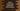# numpy.log() method explanation with examples## numpy.log():

numpy.log() is a mathematical function that is used to calculate the natural logarithm. The natural logarithm is the log value in base e.

In this post, we will learn how to use numpy.log() with examples.

### Definition of numpy.log():

Below is the definition of numpy.log():

``numpy.log(arr, /, out_arr=None, *, where=True, casting='same_kind', order='K', dtype=None, subok=True[, signature, extobj]) = <ufunc 'log'>``

Here,

• arr is the input value of type array_like.
• out_arr is an optional parameter. It can be a ndarray, None, or tuple of ndarray and None. It is the location where the output will be stored. If it is not provided, then a newly allocated array will be returned.
• where is an optional parameter of type array_like. This condition is used in the input. Where it is True, the out_arr is set to the result of ufunc. result. Else, it will be the original value. If we are not providing out_arr, the locations will be uninitialized where the condition is False.

This method returns a ndarray, the natural algorihm of the input array_like value arr.

### Example of numpy.log():

Let’s take a look at the below example:

``````import numpy as np

print('log(10) : {}'.format(np.log(10)))
print('log(e) : {}'.format(np.log(np.e)))
print('log(e**2) : {}'.format(np.log(np.e ** 2)))``````

Here, we are using log() with three different values. It will print:

``````log(10) : 2.302585092994046
log(e) : 1.0
log(e**2) : 2.0``````

We can also use it with an array:

``````import numpy as np

given_array = [2, 3.3, 4.5, 6, 10.5]
print('log for given_array : {}'.format(np.log(given_array)))``````

It will print:

``log for given_array : [0.69314718 1.19392247 1.5040774  1.79175947 2.35137526]``

We can also use it with a nd array. For example, for a two-dimensional array:

``````import numpy as np

given_array = np.array([[2, 3.3, 4.5, 6, 10.5], [1.2, 2, 3, 4, 5]])
print('log for given_array : {}'.format(np.log(given_array)))``````

It will print:

``````log for given_array : [[0.69314718 1.19392247 1.5040774  1.79175947 2.35137526]
[0.18232156 0.69314718 1.09861229 1.38629436 1.60943791]]``````